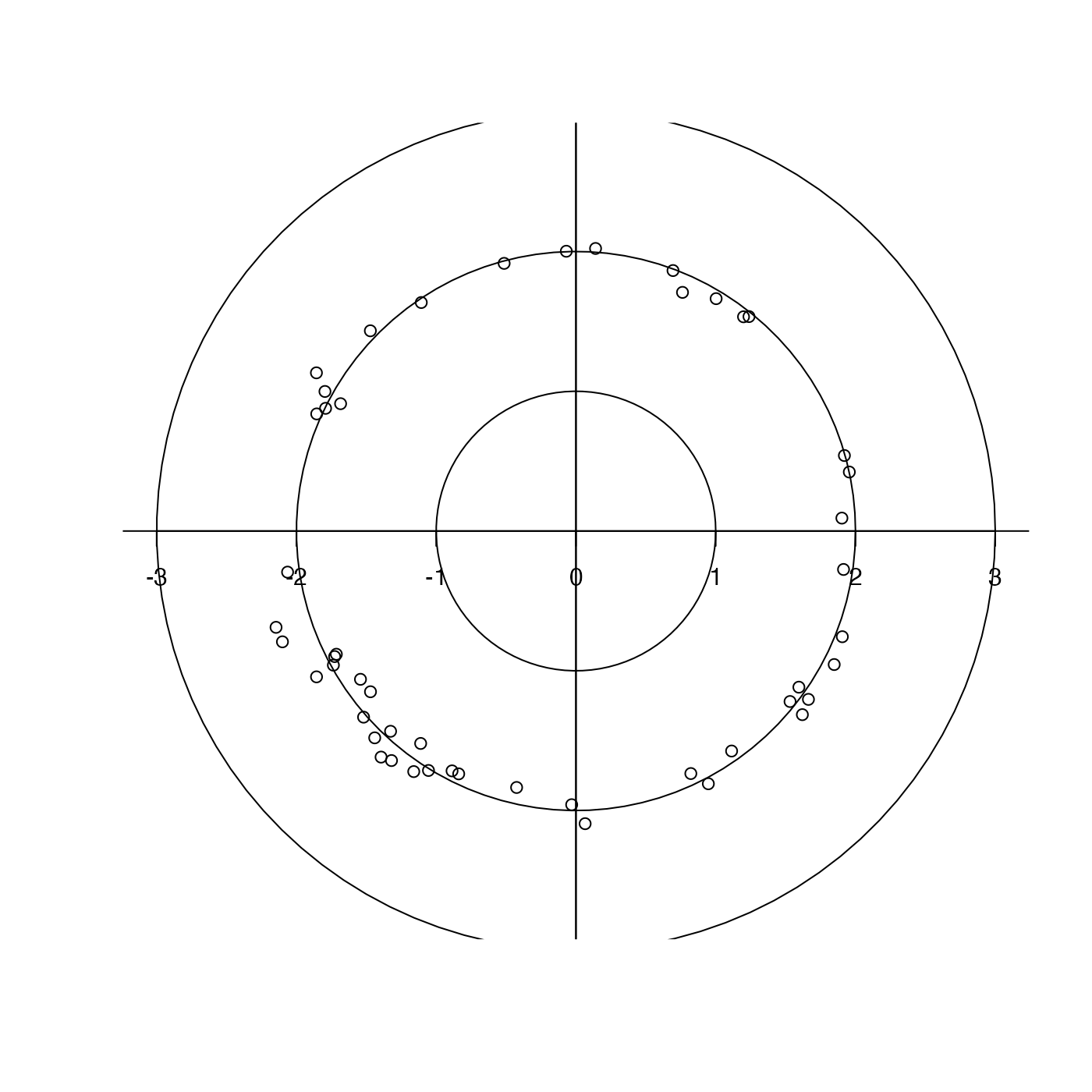Creates a crude polar plot.

plotPolar(r, theta, debug = getOption("oceDebug"), ...)

## Arguments

r radii of points to plot. angles of points to plot, in degrees. a flag that turns on debugging. Set to 1 to get a moderate amount of debugging information, or to 2 to get more. optional arguments passed to the lower-level plotting functions.

## Examples

library(oce)
r <- rnorm(50, mean=2, sd=0.1)
theta <- runif(50, 0, 360)
plotPolar(r, theta)